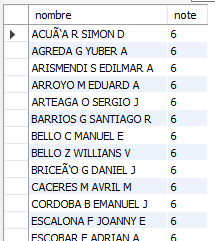# Cross Join SQL

The CROSS JOIN joins each row of the first table with each row of
the second table.

In other words, the cross join returns a cartesian product of rows from
both tables.

Unlike INNER JOIN or LEFT JOIN, CROSS JOIN does
not establish a relationship between the joined tables.

Suppose table 1 contains three rows 1, 2 and 3 and table T2 contains
three rows A, B and C.

CROSS JOIN gets a row from the first table (T1) and then creates a
new row for each row from the second table (T2). It then does the same
thing for the next row of the first table (T1) and so on.

## MySQL Server CROSS JOIN Examples

The following statement returns the combinations of all Students and
Tests.

SELECT name, note FROM alumni.ma1b02 CROSS JOIN
alumni.evaluations_notes ORDER BY note;

Obtaining the following result:As can be seen, a Cartesian product of the records in Table1 with the
records in Table2 is obtained.